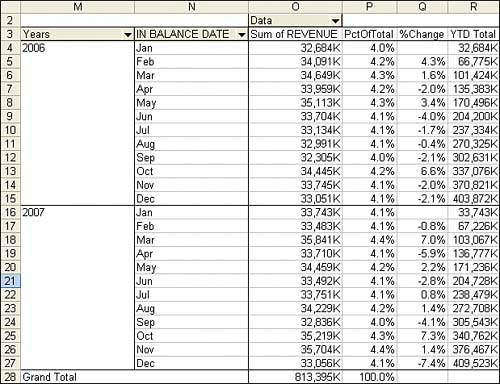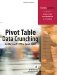# Report Percentages

In addition to the available choices, such as Sum, Min, Max, and Average, there is another set of pivot table options called the calculation options. These allow you to show a particular field as a percentage of the total, a percentage of the row, a percentage of the column, or as the percent difference from the previous or next item. All these settings are controlled through the .Calculation property of the page field.

The valid properties for .Calculation are xlPercentOf, xlPercentOfColumn, xlPercentOfRow, xlPercentOfTotal, xlRunningTotal, xlPercentDifferenceFrom, xlDifferenceFrom, xlIndex, and xlNoAdditionalCalculation. Each has its own unique set of rules. Some require that you specify a BaseField, and others require that you specify both a BaseField and a BaseItem. The following sections have some specific examples.

#### Percentage of Total

To get the percentage of the total, specify xlPercentOfTotal as the Calculation property for the page field:

` ' Set up a percentage of total With PT.PivotFields("Revenue")     .Orientation = xlDataField     .Caption = "PctOfTotal"     .Function = xlSum     .Position = 2     .NumberFormat = "#0.0%"     .Calculation = xlPercentOfTotal End With `

#### Percentage Growth from Previous Month

With ship months going down the columns, you might want to see the percentage of revenue growth from month to month. You can set this up with the xlPercentDifferenceFrom setting. In this case, you must specify that the BaseField is "In Balance Date" and that the BaseItem is something called (previous):

` ' Set up % change from prior month With PT.PivotFields("Revenue")     .Orientation = xlDataField     .Function = xlSum     .Caption = "%Change"     .Calculation = xlPercentDifferenceFrom     .BaseField = "In Balance Date"     .BaseItem = "(previous)"     .Position = 3     .NumberFormat = "#0.0%" End With `

Note that with positional calculations, you cannot use the AutoShow or AutoSort method. This is too bad; it would be interesting to sort the customers high to low and to see their sizes in relation to each other.

#### Percentage of a Specific Item

Many companies have a goal to have service revenue exceed a certain multiplier of copier sales. You can use the xlPercentDifferenceFrom setting to express revenues as a percentage of the copier product line:

` ' Show revenue as a percentage of hardware With PT.PivotFields("Revenue")     .Orientation = xlDataField     .Function = xlSum     .Caption = "% of Copier"     .Calculation = xlPercentDifferenceFrom     .BaseField = "ProductLine"     .BaseItem = "Copier Sale"     .Position = 3     .NumberFormat = "#0.0%" End With `

#### Running Total

It is not intuitive, but to set up a running total, you must define a BaseField. In this example, you have In Balance Date running down the column. To define a running total column for revenue, you must specify that BaseField is "In Balance Date":

` ' Set up Running Total With PT.PivotFields("Revenue")     .Orientation = xlDataField     .Function = xlSum     .Caption = "YTD Total"     .Calculation = xlRunningTotal     .Position = 4     .NumberFormat = "#,##0,K"     .BaseField = "In Balance Date" End With `

Figure 12.21 shows the results of a pivot table with three custom calculation settings, as discussed earlier.

##### Figure 12.21. This pivot table presents four views of Sum of Revenue. Column O is the normal calculation. Column P is % of Total. Column Q is % change from previous month. Column R is the running total.#### CASE STUDY: Special Considerations for Excel 97

Pivot tables and VBA took a radical turn in Excel 2000. In Excel 2000, Microsoft introduced the PivotCache object. This object allows you to define one pivot cache and then build many pivot reports from the pivot cache.

Officially, Microsoft quit supporting Excel 97 a few years ago. But, in practical terms, there are still many companies using Excel 97. If you need your code to work on a legacy platform, you should be aware of how pivot tables were created in Excel 97.

In Excel 97, you would use the PivotTableWizard method. Take a look at the code for building a simple pivot table showing revenue by region and line of business. Where current code uses two steps (add a PivotCache and then use CreatePivotTable), Excel 97 would use just one step, using the PivotTableWizard method to create the table:

` Sub PivotExcel97Compatible()     ' Pivot Table Code for Excel 97 Users     Dim WSD As Worksheet     Dim PT As PivotTable     Dim PRange As Range     Dim FinalRow As Long     Set WSD = Worksheets("PivotTable")     ' Delete any prior pivot tables     For Each PT In WSD.PivotTables         PT.TableRange2.Clear     Next PT     ' Define input area     FinalRow = WSD.Cells(Application.Rows.Count, 1).End(xlUp).Row     FinalCol = WSD.Cells(1, Application.Columns.Count). _         End(xlToLeft).Column     Set PRange = WSD.Cells(1, 1).Resize(FinalRow, FinalCol)     ' Create pivot table using PivotTableWizard     Set PT = WSD.PivotTableWizard(SourceType:=xlDatabase, _         SourceData:=PRange.Address, _         TableDestination:="R2C13", TableName:="PivotTable1")     PT.ManualUpdate = True     ' Set up the row fields     PT.AddFields RowFields:="Region", ColumnFields:="Line of Business"     ' Set up the data fields     With PT.PivotFields("Revenue")         .Orientation = xlDataField         .Function = xlSum         .Position = 1         .NumberFormat = "#,##0,K"         .Name = "Total Revenue"     End With     PT.ManualUpdate = False     PT.ManualUpdate = True End Sub `Pivot Table Data Crunching for Microsoft Office Excel 2007
ISBN: 0789736012
EAN: 2147483647
Year: 2003
Pages: 140

Similar book on Amazon